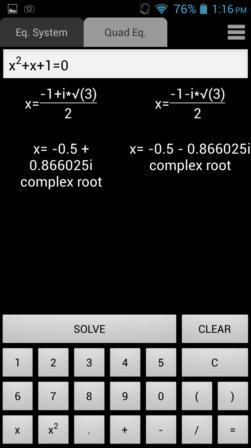Editor Ratings:
User Ratings:
[Total: 0 Average: 0]

Here’s a list of 5 equation solver apps for Android. With these apps you’ll be able to solve equations more easily. Equations are causing a lot of problems to a lot of students around the world. With the equation solver apps mentioned down below you might not be able to solve more complex equations, but they can still be useful to check if your own solutions of more simplistic equations are correct. Note that most of the apps don’t show the actual procedure of how equations are solved, just the end result, so they won’t help you cheat.

##(adsbygoogle = window.adsbygoogle || []).push({});

Equation Solver is an equation solver app which supports all the basic mathematical functions, multiplication, division, addition, subtraction, trig functions, square roots, logarithms and absolute values.

App doesn’t have pre-configured formulas that you can use, instead you type in the equation into the app after which it will go through all the possible solutions and present the one that make sense to you. There’s a helpful tutorial after running the app for the first time that can help you understand how the app works.

Get Equation Solver.

##yHomework – Math Solver has a much intuitive interface than Equation Solver. What you see on the image above is the solution for a quadratic equation that I typed into the app.

Design of the app simulates a “pen on paper” experience. All the already mentioned math functions are supported, plus notice that with yHomework you actually get the entire explanation where it’s described how the equation needs to be solved step by step. Currently, the app covers simplification, single unknown equation, two equation sets and the already mentioned quadratic equations.

Also have a look at Math Solver: Free Windows 8 App To Solve Math Problems.

##(adsbygoogle = window.adsbygoogle || []).push({});

GES Equation Solver is a very simple and lightweight math equation solver which is just a couple of MB in size.

Math equations and functions mentioned up until now are also available in GES Equation Solver. What makes it interesting, and what makes it pop is that it has a description explaining each step of the equation solving process, see image above. Solution can be easily emailed via Gmail from within the app.

##Equation System Solver is an equation solver app for Android that’s aimed specifically at equation systems and quadratic equations. Notice the two tabs in the top left corner of the screen.

Up to five equations can be typed into the app. To type in the quadratic equation switch to the second tab and type it into the field at the top. Only the solutions are offered unfortunately, there’s no step by step guide.

Get Equation Solver.

Also have a look at Free Boolean Logic Equation Solver: Simple Solver.

##Equation Solver is another simplistic, but very feature advanced equation solver which supports functions like basic arithmetic operations, trigonometric operations, logarithms, etc.

Using these functions you can setup lots of different types of mathematical equations for the app to solve.

Get Equation Solver.

## Conclusion

From the list above I would have to say that yHomework and GES Equation solver are my favorites. They are my favorites because both of them have step by step directions and description for how to solve equations.

 Editor Ratings: User Ratings:[Total: 0 Average: 0] Works With: Android Free/Paid: Free